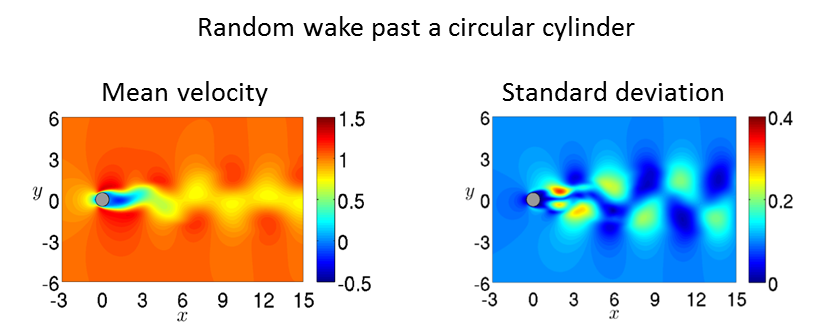Fundamentals of Uncertainty Quantification in Computational Science and Engineering

Course Description: Computing the statistical properties of nonlinear random systems is of fundamental importance in many areas of science and engineering. The primary objective of the course is to introduce students to state-of-the-art methods for uncertainty propagation and quantification in model-based computations, focusing on the computational and algorithmic features of these methods most useful in dealing with systems specified in terms of stochastic ordinary and partial differential equations. The heightened relevance of UQ in computational science and engineering has recently driven an explosive growth of fundamental and practical developments at the interface of high-performance scientific computing, probability theory, and applied mathematics. Topics covered include: polynomial chaos methods (gPC and ME-gPC), probabilistic collocation methods (PCM and ME-PCM), Monte-Carlo methods (MC, quasi-MC, multi-level MC), sparse grids (SG), probability density function methods, and techniques for dimensional reduction. Basic knowledge of probability theory and elementary numerical methods for ODEs and PDEs is recommended.

The course will focus primarily on the so-called forward UQ problem, in which uncertainties in input parameters such as initial conditions, boundary conditions, geometry or forcing terms are propagated through the equations of motion of the system into their solution. This includes nonlinear models of classical physics such as fluid dynamics systems governed by the Navier-Stokes equations, and models of system biology described by systems of stochastic ordinary differential equations.The inverse UQ problem, on the other hand, addresses fundamental questions such as model inadequacy, as well as calibration and validation of models built upon incomplete information. The natural setting for the inverse UQ problem is Bayesian.

Instructor: Prof. Daniele Venturi

Main Lectures: MWF 1:20-2:25PM, BE-169

Office Hours: Thursday 9:30-11:30AM,  BE-353B

LECTURE NOTES: PDF

Final Presentations: Monday March 18, room and time TBD

Syllabus

• Week 1: Elements of probability theory; random variables, random vectors, stochastic processes, random fields and their representation.
• Week 2: Introduction to uncertainty quantification (UQ): objectives, model equations and probabilistic framework. Forward and inverse UQ problems; examples in systems biology, fluid dynamics and other disciplines.
• Week 3: Monte-Carlo methods for the solution of stochastic ordinary and partial differential equations; quasi-MC, low-discrepancy sequences, multi-level MC.
• Week 4: Polynomial chaos: theory of orthogonal polynomials and approximation theory, generalized polynomial chaos (gPC); Multi-element generalized polynomial chaos (ME-gPC); Stochastic Galerkin method.
• Week 5-6: Probabilistic collocation method; multi-element probabilistic collocation; tensor product collocation; sparse grid collocation , Smolyak algorithm, adaptive Leja rules; discrete projection (pseudo-spectral approach); stochastic Galerkin versus probabilistic collocation methods.
• Week 7: Dynamically orthogonal and bi-orthogonal field equation methods; applications to nonlinear PDEs.
• Week 8: Generalized spectral decomposition for nonlinear stochastic problems; computational algorithms (alternating direction Galerkin methods, power methods)
• Week 9-10: Probability density function (PDF) methods; nonlinear dynamics and Liouville equations; solution methods; dynamical systems driven by colored random noise, Fokker-Plank and effective Fokker-Plank equations; solution methods. Dimension reduction; hierarchies of PDF equations (BBGKY and Lundgren-Monin-Novikov hierarchies), closure approximations. PDF methods in fluid dynamics. Functional differential equations (Hopf functional equations, probability density functional equations).

Students will be evaluated on the basis of:

1. Homework assignments
2. Final research project related to the course material

The final project can be an original research paper on an in-depth report on an existing published paper. In the latter case, the students are required to reproduce all analytical and numerical results in the paper. The final projects have two components: a piece of work (paper) handed in before the last day of class, and an oral presentation which will take place during finals week.